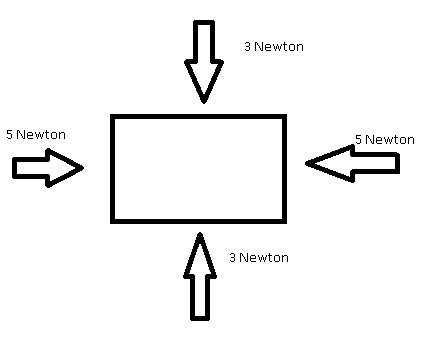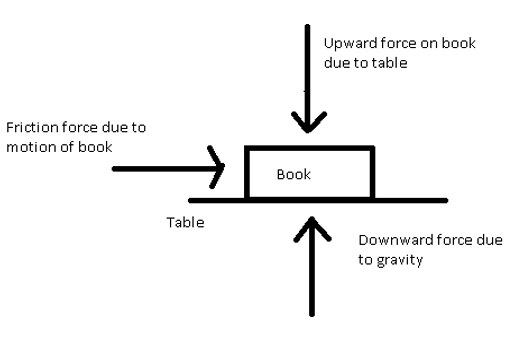# Define balanced and unbalanced force

## Introduction

Balanced and Unbalanced forces decide the state of an object. In simple language, Force is the interaction between two objects. It is a push or pulls that changes its position and causes the change in velocity. It is capable of doing work to change the state (rest state or motion state) of a particular body.

Force is a vector quantity so that it has magnitude and direction. If two forces are applied to a body then it is called balance force If both forces have equal magnitude and are opposite in direction. So that if the net force acting on the body is zero then it is said to be a balanced force. And if the net force acting on a body is not zero then it is called unbalanced force. When balance force acts on the system then it is in equilibrium condition.

## What are Balanced Forces?

Balanced forces are the forces that exert on the body or system in equal magnitude and they are acted in opposite directions. Under balanced forces, the net force on the system is zero. So that the body is at rest and it is called equilibrium and it has zero acceleration. Balance forces neither change the state of rest nor the state of motion.

Example: Let's consider four forces acting on the rectangular box the below diagramFrom the diagram, there is an upward force of 3 Newton and a downward force of 3 Newton so they cancel each other and there is no net force in the Y-direction and in the X-direction, both forces have the same magnitude which is 5 Newton, and in opposite directions so forces are balanced in the x-direction also. Therefore, this rectangular box has under balanced forces.

## Examples of Balanced Forces

### Pushing a Wall

When you push a wall and it does not move then it indicates both the wall and man have applied the same force on each other.

### Standing a man on the Ground

When you are standing on the ground, a gravitational force acts on man downwards and a normal force act on man in the upward direction both forces have the same magnitude so that they cancel out each other and man remains at rest.

### Tug of war in which Teams have equal members

In the game of tug of war members of one team pull another team so that they applied force on others team members. If there are equal members in both teams then each team has applied the same force on another team, So, that there is a net force of zero and the system is in balance or equilibrium.

### Book on the Table

When a book is placed on a table it is under balance force because equal force acted on it in the opposite direction.

## Characteristics of Balanced Force

• Under balanced forces motion of the body does not change.

• When the system experiences a balancing force there is no charge in state and direction.

• The system is in static equilibrium under balanced forces.

• The net force on the system is zero.

## Effect of a Balanced Force on a Body

When forces acting on a body are balanced then there is zero resultant force on the body so balanced force does not change the state of the body. It doesn't make a body move if it is at rest and if the body is in motion, then it continues its motion under the balancing force.

## What are Unbalanced Forces?

A force is said to be an unbalanced force if it changes the motion of a body. If the body is at rest, then we can make the body move by applying unbalanced force. Same as if the body is in motion, then we can stop it or make it at a state of rest by using unbalanced force. When two forces of unequal size are acted on the body then the system is under unbalanced force. The resultant force on a body is not zero so there is non-zero acceleration and does not have an equilibrium condition.

Example: We can understand the unbalanced force by an example. Let's assume a book is placed on the table and due to the inclination of the table it moves towards the right.An upward force acting on the book because of the table and a downward force due to gravity because there is no motion in the vertical direction. These forces balance each other. Due to the motion of the book in the right direction, there is friction force in the left direction which opposes the motion and slows down the speed of the book. The book changes its state of motion with time and not equilibrium so the motion of the book is considered under unbalanced force.

## Examples of Unbalanced Forces

### Tug of war in which teams have unequal members

In the game of tug of war members of one team pull another team so that they applied force on other team members. If there are unequal members in both teams then each team has applied the force on another team, and forces are not the same So that there is the net force in the system and there is the acceleration of the system.

### See saw the Game

In this game if two people of unequal weight have placed the see-saw game then it is under the unbalanced force.

### Kicking a Ball

When the ball is kicked it travels from one place to another hence it can be considered an example of an unbalanced force.

## Difference between Balanced and Unbalanced Forces

S.No.Balanced ForceUnbalanced Force
1.Balanced force does not produce any change in the motion of the body .Unbalanced force changes the motion of the body.
2.The net effective force on the system is zero if balance forces are there. So, no acceleration is occurring in the system.The net effective force on a system is not zero if unbalanced forces are there it produces the acceleration in the system.
3.It can only change the shape or size of the object and cannot change the motion of the object.It can not only change the shape or size but also can change the motion of the object.

Table-1: Difference between balanced and unbalanced forces

## Conclusion

In this article we have understood the concept of forces and the main types of forces that are balanced or unbalanced forces. Balanced forces do not change the state of any object but the unbalanced forces are the reason for the motion of the body.

The net acceleration and force on the body under balanced forces are zero. Whereas net acceleration occurs in the system due to unbalanced forces. So, we learned that unbalanced forces are responsible for the occurrence of any type of motion. Friction is a type of force that can resist the motion of the particle and acts between the contact surface of the two bodies. Unbalanced force can change the shape as well as the state of motion.

## FAQs

Q1. Write down the names of the state of motion?

Ans. There are two types of state of motion. One is the rest state of motion in which the system is in static equilibrium and another is the state of motion in which the system is moving.

Q2. Can a balanced force deform a body?

Ans. Yes, a balanced force can deform a body or can change the shape or size of the body. But never change the state of motion of the body.

Q3. What is the formula of force when acceleration is given?

Ans. Force is the multiplication of mass of the body and acceleration of the body.

$$\mathrm{F=ma}$$

Here m is the mass of the body and a is acceleration of the body.

Q4. What is the unit of force?

Ans. S.I. unit of the force is newton or $\mathrm{kgms^{-2}}$.

And C.G.S. unit of the force is dyne or $\mathrm{gm\: cm \:s^{-2}}$

Q5. Give one example to prove that balanced forces are used in daily life?

Ans. Screws or clips which are hooked to the wall for hanging the things are examples of balanced force in which gravitational force is balanced by the force which attaches the hook to the wall.

Updated on: 28-Apr-2023

110 Views